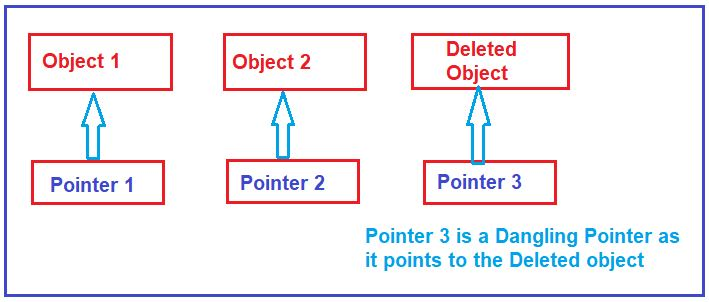# Dangling Pointer in C

## Dangling Pointer in C Language with Examples

In this article, I am going to discuss Dangling Pointer in C Language with Examples. Please read our previous articles, where we discussed Void Pointer in C Language with Examples.

##### Dangling Pointer in C Language:

The most common bugs related to pointers and memory management in C Programming Language is Dangling/Wild Pointers. Sometimes as a programmer, we forgot to initialize the pointer with a valid address, and when we do so, then this type of uninitialized pointer is known as a dangling pointer. Dangling pointer occurs at the time of the object destruction when the object is deleted or de-allocated from memory without modifying the value of the pointer. In this case, the pointer is pointing to the memory, which is de-allocated.

The pointer variable which is pointing to an inactive or dead memory location is called Dangling Pointer. Or in other words, we can say that a pointer pointing to a memory location that has been deleted (or freed) is called a dangling pointer in the C language. For a better understanding, please have a look at the below diagram.In the above diagram, you can see we have three-pointers and observe that Pointer 3 is a dangling pointer. Pointer 1 and Pointer 2 are the pointers that point to the allocated objects, i.e., Object 1 and Object 2, respectively whereas Pointer 3 is a dangling pointer as it points to a deleted object.

##### How to Create Dangling Pointer in C Language?

In C Programming Language, there are three different ways where we can make a pointer acts as a dangling pointer. They are as follows:

• De-allocation of memory
• Function Call
• Variable goes out of scope
##### Creating Dangling Pointer by De-Allocating the Memory:

Deallocating a memory that is pointed by a pointer causes a dangling pointer. In the below example, we have created an integer pointer. Then we call the free function by passing the pointer. Once we call the free function, then the pointer ptr becomes a dangling pointer.

```#include <stdlib.h>
#include <stdio.h>
int main()
{
int *ptr = (int *)malloc(sizeof(int));
free(ptr);
}```
##### Avoiding Dangling Pointer Errors in C Language:

In C Programming Language, the dangling pointer errors can be avoided by initializing the pointer to a NULL value. If we assign the NULL value to the pointer, then the pointer will not point to the de-allocated memory instead the pointer is not pointing to any memory location. So, in the below example, after calling the free function, we initialize the pointer with a NULL value and hence it is not a Dangling Pointer anymore.

```#include <stdlib.h>
#include <stdio.h>
int main()
{
int *ptr = (int *)malloc(sizeof(int));
free(ptr);
ptr = NULL;
}```
##### Creating Dangling Pointer by Function Call:

In C Language, the pointer pointing to the local variable becomes dangling when the local variable is not static. In the below example, the variable x is a local variable and goes out of scope once the execution of the fun() function is completed. And inside the main method, the pointer p is pointing to something which does not exist anymore and hence the pointer ptr becomes a dangling pointer.

```#include<stdio.h>
int *fun()
{
int x = 5;
return &x;
}

int main()
{
int *ptr = fun ();
printf ("%d", *ptr);
return 0;
}```

In the above program, ptr is called a Dangling Pointer because according to the storage class of C, by default any type of variable storage class specifier is auto, and the lifetime of the auto variable is within the body only. But in the program, the control is passed back to the main function and still, the pointer is pointing to that inactive variable only.

The solution of the dangling pointer is in place of creating an auto variable, create a static variable because the lifetime of the static variable is the entire program. Let us modify the program as follows where we make the variable x static. Once we make variable x static, now the variable x has scope throughout the program. So, the point that you need to remember is, that the pointer pointing to the local variable doesn’t become a dangling pointer when the local variable is static.

```#include<stdio.h>
int *fun()
{
static int x = 5;
return &x;
}

int main()
{
int *ptr = fun ();
printf ("%d", *ptr);
return 0;
}```

Output: 5

##### Creating Dangling Pointer by Variable goes out of scope:

When the variable goes out of scope, then the pointer becomes a dangling pointer. In the below example, first, we created one pointer ptr. Then with the variable scope, we initialized the pointer. Once the variable goes out of scope, then it becomes a dangling pointer

```#include<stdio.h>
void main()
{
int *ptr;
//Variable scope started
{
int ch;
ptr = &ch;
}
//Variable scope ended
// Now ptr is dangling pointer
printf ("%d", *ptr);
return 0;
}
```